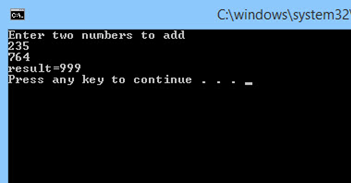# Batch File Variables

Any programming or scripting languages require variable to store the data and access them whenever we need the value. Using of variables becomes inevitable in mathematical computations. The variable contains some data whose value may change during the course of program computation.If you are familiar with traditional languages like C, C++ or java, you may assume that the variable must be created generally using the data type that defines the value it holds. But in batch files we don’t have any such data type like int, float blah blah. The command interpreter is intelligent enough to understand certain values given to variables.

Let us first understand how to create variables in batch file programming and go deeper into this concept.

## Batch File Variables

So, the above syntax is used to evaluate arithmetic expressions and also create variables in batch programs. Basically we create variables to specify that the computer needs to remember certain values that are given a name and may be changed at any time until the end of the program.

Does this syntax seems weird?

Yes, I felt the same at first. If you are a novice programmer, you will also feel the same. Let us break it down and make it simple enough to understand. For few minutes, forget the syntax and just remember that we use SET command to evaluate arithmetic expressions and create variables.

## Batch File SET Variable

Just we will start with the first word in the syntax that is SET. This word is enough for us to create a variable. It is a built in command in MS-DOS. Now the question is how to create a variable using this command. Have a glance at the example.

### Creating Variables

This line creates a variable named myVar with its value as 1. The variable name is myVar and its value is an integer (Literally to say the interpreter doesn’t know that myVar holds an integer. You will know why as we move on further.)

I can use either a number or a string in the place of value like as shown below.

Like all other languages, we also have certain restrictions on the variable name that the names must not be built in commands etc. You just need to follow them while creating a variable.

### How to Print Variables in Batch File?

To print a message on the command prompt we already know that we use ECHO command.

Will “Echo myVar” prints the values of the variable on the console?

No, the interpreter doesn’t know that by writing myVar, you are referring to a variable. But, it assumes that myVar is a string that needs to be echoed back on the console. So, to tell it is a variable, we enclose it in ‘%’ signs.

Program #1

The above program prints the value of myVar on the command prompt. Give it a try and observe it. If we want to refer a variable that already exists before, we enclose the variable name in ‘%’ signs.

Now let us write a program to carry out addition of two variables.

So, you will be away to think that the value of the variable ‘res’ is 3. But that is not the case here. The interpreter assumes res as a string that is the concatenation of variables a and b. To specify that the computation is arithmetic but not the string concatenation, we use the flag ‘/a’. Using it, let us rewrite the program.

Now the resultant value in ‘res’ will be 3.

How to save the user inputs in a variable?
Immediately after the above program, you might be interested to get the variable values from the user rather than making a static calculation.

For this purpose, we use ‘/p’ flag. ‘/p’ flag is used to set the value of variable taken from the line of input. We will design a simple calculator that adds two numbers given by the user to make it clearer.

### Batch Program for Addition of Two Numbers

First input given from the user will be stored in ‘num1’ and the next in ‘num2’. Then we carry out the addition and display the result. The output window is shown below.More fun to experiment yourself
1. Write a batch file to add, multiply, divide and subtract two numbers. Observe the results.

2. Use ‘goto’ statement in the above program to repeat the addition of two numbers any number of times. (If you don’t get this, nothing is there to worry. In the next tutorial, I’ll explain how about looping and conditional statements).

This was all about batch file variables. If you have any doubts then you can ask it by commenting below.

### 4 thoughts on “Batch File Variables”

1.Hi I’m trying this out but the command window result doesn’t print the variable it just ends with “result = ” ..this is the code – I’m using windows 10:

echo Enter two numbers to add:
SET /p num1=
SET /p num2=
SET a/ res=%num1%+%num2%
echo result^=%res%
Pause

2.SET a/ res=%num1%+%num2%
syntax error in above
it should be…..SET /a res=%num1%+%num2%

3.use this SET a/ res=%num1%+%num2%
it will work.

4.Trying to make a batch that requests the crumb for Jenkins and then uses that crumb returned in the next command. Here is a sample of the two.